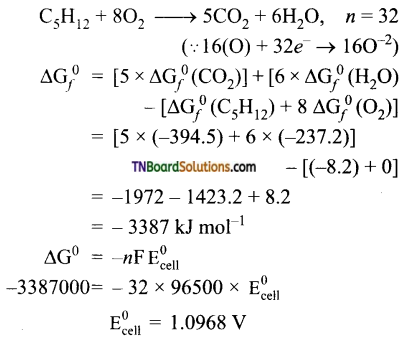Students get through the TN Board 12th Chemistry Important Questions Chapter 9 Electro Chemistry which is useful for their exam preparation.

## TN State Board 12th Chemistry Important Questions Chapter 9 Electro Chemistry

Question 1.
Define the following terms:
(i) Resistivity,
(ii) conductivity,
(iii) cell constant,
(iv) specific conductance,
(iv) molar conductivity,
(vi) equivalent conductance.
(i) Resistivity is defined as the resistance of an electrolyte confined between two electrodes having unit cross-sectional area and are separated by unit distance.
(ii) The reciprocal of specific resistance $$\left(\frac{1}{\rho}\right)$$ is called specific conductance or conductivity.
(iii) The ratio between the distance between the two electrodes in a conductivity cell to their area of cross-section is called cell constant.
(iv) The specific conductance is defined as the conductance of a cube of an electrolytic solution of unit dimension.
(v) Molar conductance is defined by the conducting power of all the ions produced by 1 mole of the electrolyte.
(vi) Equivalent conductance is defined as the conductance of ‘V’ m3 of the electrolyte solution containing one gram equivalent of electrolyte in a conductivity cell in which the electrodes are 1 meter apart.

Question 2.
Give the relationship between resistance and specific resistance of an electrolytic conductor.
R = ρ × $$\frac{l}{a}$$
where ‘R’ is the resistance, ρ is the specific resistance or resistivity, ‘l’ is the distance between the electrodes in a conductivity cell, and ‘a’ is the area of cross-section of electrodes.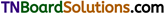Question 3.
Mention the units of (i) resistance, (ii) specific resistance, (iii) conductance, (iv) specific conductance, (v) molar conductance and (vi) equivalent conductance.

 Property Unit Resistance ohm Specific resistance ohm metre (Dm) Conductance Siemens (s) Specific conductance ohm-1m-1 or mhom-1 (Sm-1–) Molar conductance Sm2mol-1 Equivalent conductance Sm-1

Question 4.
Give the relationship between molar conductance and specific conductance of an electrolyte.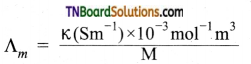Question 5.
Give the relationship between equivalent conductance and specific conductance of an electrolyte.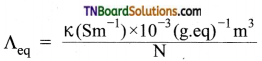Question 6.
Mention the factors which influence electrolytic conductance.

1. A solvent of a higher dielectric constant shows high conductance in solution.
2. Conductance is inversely proportional to the viscosity of the medium, i.e., conductivity increases with the decrease in viscosity.
3. If the temperature of the electrolytic solution increases, conductance also increases. An increase in temperature increases the kinetic energy of the ions and decreases the attractive force between the oppositely charged ions and hence conductivity increases.
4. Molar conductance of a solution increases with an increase in dilution. This is because, for a strong electrolyte, interionic forces of attraction decrease with dilution. For a weak electrolyte, the degree of dissociation increases with dilution.Question 7.
Explain how does the molar conductance of the electrolytes varies with the concentration of the electrolyte.
The molar conductance of a strong electrolyte increases on dilution. The variation of molar conductance with dilution is given by an empirical formula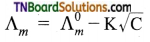where Λm0 is called limiting molar conductivity and C is the concentration of the electrolyte.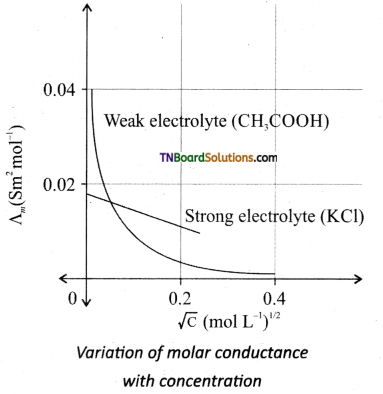For strong electrolytes such as KCl, NaCl, etc., the plot, Λm vs √C, gives a straight line as shown in the graph. It is also observed that the plot is not a linear one for weak electrolytes.
For strong electrolyte, at a high concentration, the number of constituent ions of the electrolyte in a given volume is high and hence the attractive force between the oppositely charged ions is also high. Moreover, the ions also experienced a viscous drag due to greater solvation. These factors attribute to the low molar conductivity at high concentrations. When the dilution increases, the ions are far apart and the attractive forces decrease. At infinite dilution the ions are so far apart, the interaction between them becomes insignificant, and hence, the molar conductivity increases and reaches a maximum value at infinite dilution.
For a weak electrolyte, at high concentration, the plot is almost parallel to the concentration axis with a slight increase in conductivity as the dilution increases. When the concentration approaches zero, there is a sudden increase in the molar conductance and the curve is almost parallel to Λm0 axis. This is due to the fact that the dissociation of the weak electrolyte increases with the increase in dilution (Ostwald dilution law). Λm0 values for strong electrolytes can be obtained by extrapolating the straight line. But the same procedure is not applicable for weak electrolytes, as the plot is not a linear one, A°m values of the weak electrolytes can be determined using Kohlraush’s law.

Question 8.
Flow much charge is required for the following reactions?
(i) 1 mole of Al3+ to Al
(ii) 1 mole of Cu2+ to Cu
(iii) 1 mole of MnO4 to Mn2+
(i) Al3+ +3e → Al
Reduction of 1 mole of Al3+ requires 3 moles of electrons.
∴ Charge on 3 moles of electrons
= 3 × 96500 C
= 289500 coulomb
(ii) Cu2+ + 2e → Cu
Reduction of 1 mole of Cu2+ requires 2 mole of electrons
= 2 × 96500 C
= 193000 coulomb
(iii) MnO4 + 8H+ + 5e → Mn2+ + 4H2O
Reduction of MnO4 ions require 5 mole of electrons
= 5 × 96500 C
= 482500 CoulombQuestion 9.
A solution of CuSO4 is electrolyzed for 10 minutes with a current of 1.5 amperes. What mass of copper deposited at the cathode?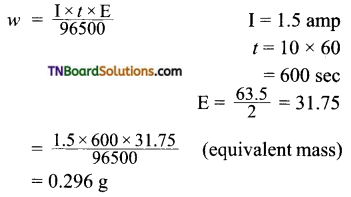∴ Mass of copper deposited at the cathode = 0.296 g

Question 10.
How many hours does it take to reduce 3 mole of Fe3+ to Fe2+. With 2 ampere of current? (F = 96500 coulomb)
The required equation is Fe3+ + e → Fe2+
3 mole of Fe3+ requires 3 mole of electrons.
∴ Required charge = 3 × 96500 coloumbs
= 289500 coloumbs
charge = current × time
289500 = 2 × t
t = $$\frac{289500}{2}$$
= 1.475 × 105 sec
= 40 hour.

Question 11.
In an electrolysis experiment, a current was passed for 5 hours through two cells connected in series. The first cell contains a solution of gold salt and the second cell contains copper sulfate solution.
If the oxidation number of gold is +3, find the amount of copper deposited on the cathode in the second cell. Also, calculate the magnitude of the current in amperes.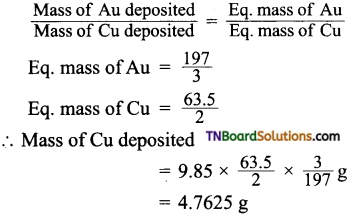Let Z be the electrochemical equivalent of copper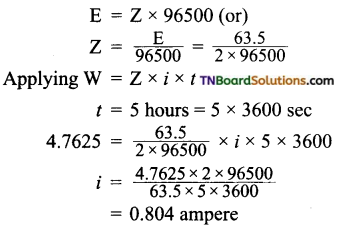Question 12.
The specific conductance of a decinormal solution of KCl is 0.00112 ohm-1 cm-1. The resistance of a cell containing the solution was found to be 56 ohms. What is the value of the cell constant?
Specific conductance = $$\frac{Cell constant}{Resistance}$$
Cell constant = Specific conductance × Resistance
= 0.0112 × 56
= 0.6272 cm-1Question 13.
1.0 N solution of salt surrounding two platinum electrodes 2.1 cm apart and 4.2 sq.cm in area was found to offer a resistance of 50 ohms. Calculate the equivalent conductivity of the solution.
Given: l = 2.1 cm; a = 4.2 sq.cm; R = 50 ohm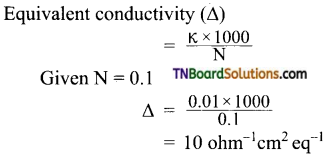Question 14.
The specific conductivity of 0.02 M KCl solution at 25° C is 2.768 × 10-3 ohm-1 cm-1. The resistance of this solution at 25° C, when measured with a particular cell, was 250.2 ohm. The resistance of 0.01 M CuSO4 solution at 25° C measured with the same cell was 8331 ohm. Calculate the molar conductivity of the copper sulfate solution.
Cell constant = Specific conductivity of KCl × Resistance = 2.768 × 10-3 × 250.2
For 0.01 M CuSO4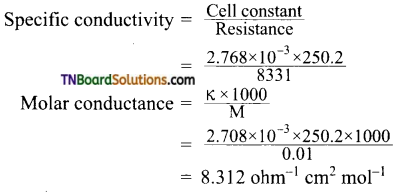Question 15.
At 291 K, the molar conductivities at infinite dilution of NH4Cl, NH4OH, and NaCl are 129.8, 217.8, and 108.9 S cm2 respectively. If the molar conductivity of a cent normal solution of NH4OH is 9.33 S cm2, what is the percentage dissociation of NH4OH at this dilution? Also, calculate the dissociation constant of NH4OH.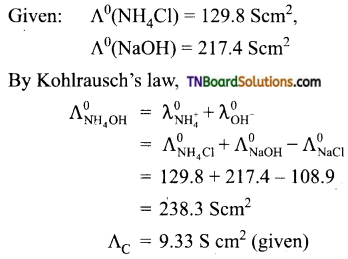∴ degree of dissociation (α)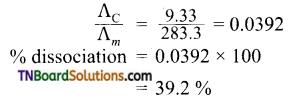Calculation of dissociation constant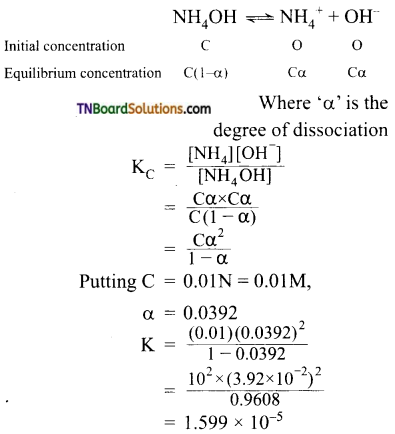Question 16.
The conductivity of a saturated solution of AgCl at 288 K. is found to be 1.382 × 10-6 Scm-1. Find its solubility. Given ionic conductances of Ag+ and Cl ion at infinite dilution are 61.9 S cm2 mol-1 and 76.3 S cm2 mol-1 respectively.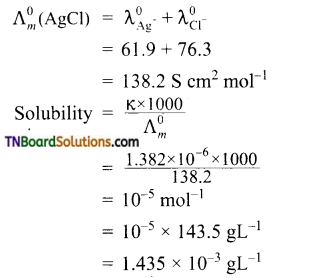Question 17.
Define (i) electrode potential and (ii) emf of a cell.
(i) Electrode potential: The potential difference set up between the metal and its ions in the solution is called the electrode potential, (or) electrode potential may be defined as the tendency of an electrode to lose or gain electrons when it is in contact with a solution of its own ions.
(ii) emf of a cell; The difference between the electrode potentials of the two half cells in a galvanic cell is known as the cell potential (or) It is the driving forces that permit the flow of electrons from anode to cathode.

Question 18.
Explain the terms (i) oxidation potential and (ii) reduction potential of an electrode.
(i) Oxidation potential: when an electrode is negatively charged with respect to the solution, i.e., it acts as an anode, the potential difference is called oxidation potential.
M ⇌ Mn+ + ne
It refers to the tendency for oxidation to occur at the electrode.
(ii) Reduction potential: When an electrode is positively charged with respect to solution, i.e., it acts as the cathode, the potential difference developed is called reduction potential
Mn+ + ne ⇌ M
It refers to the tendency for reduction to occur at the electrode.

Question 19.
What is a reference electrode? Mention its use.
A reference electrode is an electrode whose electrode potential value is known. It is used to determine, electrode potentials of various electrodes.

Question 20.
Mention the characteristics of electrode chemical series.

1. The negative sign of the standard reduction potential indicates that the electrode when joined with SHE, acts as an anode and oxidation occurs at this electrode. Similarly, the sign of standard reduction potential indicates that the electrode when joined with SHE acts as and reduction occurs on this electrode.
2. Greater the value of standard reduction potential move easily the substance (element or ions) is reduced or in other words, stronger oxidizing agent it is. Similarly, the lower the value of standard reduction potential of the substance greater is the extent of oxidation half-reaction and the substance acts as a reducing agent.
3. Reactivity of metals: A metal that has a high negative value (or small positive value) of standard reduction potential loses electrons is said to be chemically active. The chemical reactivity decreases from top to bottom of the series.Question 21.
Given the standard reduction potentials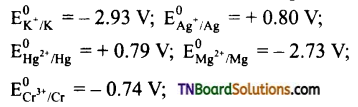Arrange these metals in their increasing order of reducing character.
Ag < Hg < Cr < Mg < K.
The lesser the reduction potentials greater is their reducing character.

Question 22.
Write down the electrode reactions and the net cell reaction for the following cells. Which electrode would be the positive terminal in each cell.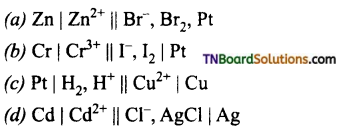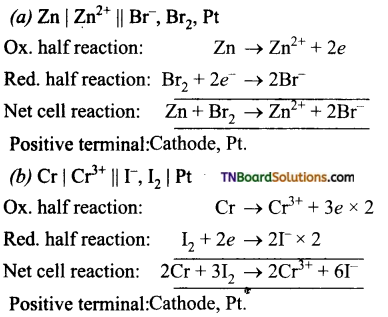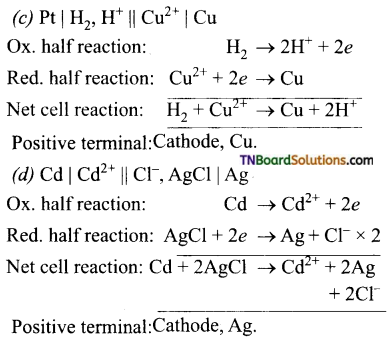Question 23.
The standard EMF of the cell:
Ni | N12+ || Cu2+ | Cu is 0.59 V. The standard reduction potential of the copper electrode is 0.34 V. Calculate the standard reduction potential of the nickel electrode.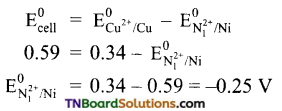Question 24.
A cell is set up between copper and silver electrodes as Cu | Cu2+ || Ag+ | Ag.
Given: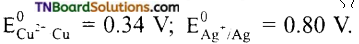. Calculate the emf of the cell.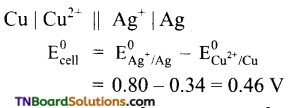Question 25.
Two half cells are Al3+ | Al and Mg2+ | Mg. The reduction potentials of these half cells are -1.66 and -2.36 V. Construct the galvanic cell, write the cell reaction and calculate the emf of the cell.
For the construction of a galvanic cell,
= (Electrode with lesser value of reduction potential – Electrode with higher value of reduction potential )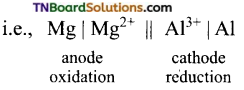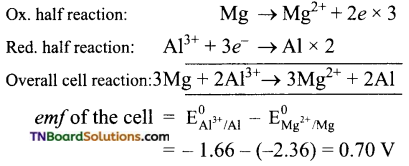Question 26.
A cell is prepared by dipping a copper rod in 1M CuSO4 solution and a nickel rod in 1M NiSO4 solution. The standard reduction potentials of copper and nickel electrodes are 0.34 V and – 0.25 V respectively.
(a) How will you represent the cell?
(b) What will be the cell reaction?
(c) What will be the standard emf of the cell?
(d) Which electrode will be positive?
The cell is represented as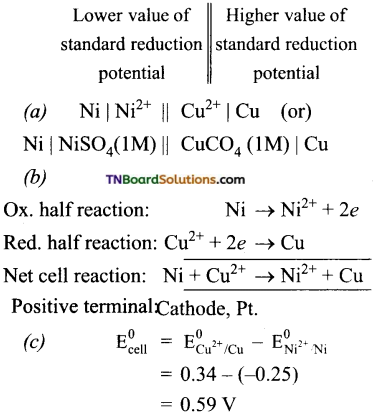(d) The cathode is a copper electrode. Because reduction occurs at this electrode.Question 27.
Predict whether zinc and silver react with 1M H2SO4 to give hydrogen gas or not. Given the standard reduction potentials of zinc and silver electrodes are -0.76 and 0.80 V respectively.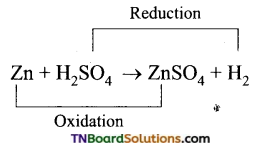The cell is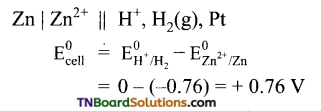The emf of the cell is positive. Hence the cell reaction is spontaneous. Thus, zinc will liberate hydrogen gas from 1M H2SO4.
Similarly,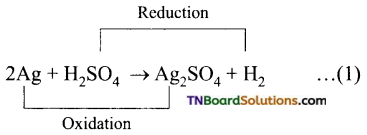The cell is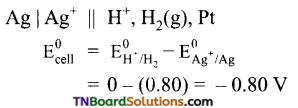The emf of the cell is negative. Hence the reaction will not occur, i.e., Ag will not displace hydrogen from 1M H2SO4.

Question 28.
Can a nickel sulphate be used to stir a solution of copper sulphate. Support your answer with a reason.
[OR]
Can a solution of 1M copper sulphate be stored in a vessel made of nickel.
Given: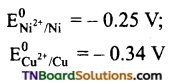The reaction,
Ni + CuSO4 → NiSO4 + Cu
should not occur, if CuSO4 solution to be stored in a nickel vessel.
The cell would be Ni | Ni2+ || Cu2+ | Cu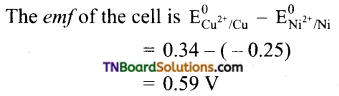The emf is positive. This means copper sulfate reacts with nickel, Hence CuSO4 cannot be stored in a nickel vessel.Question 29.
Give the relationship between the electrode potential and the concentration of the electrode.
This relationship is given by Nernst equation. For an electrode reaction,
Mn+ + ne → M
the electrode potential is given by the relationship,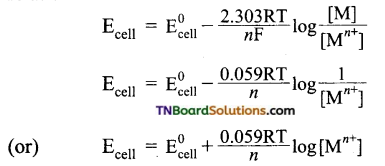Question 30.
For general cell reactions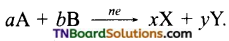Write the Nernst equation.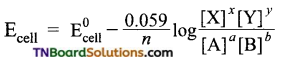where is the number of electrons involved in the cell reaction?

Question 31.
Calculate the electrode potential of copper electrode, when a copper wire is dipped in 0.1 M CuSO4 solution ECu2+/Cu0 = 0.34.
The electrode reaction is Cu2+ + 2e → Cu
Applying Nernst equation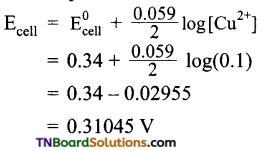[Note: Decreasing concentration of the electrolyte decreases the electrode potential]

Question 32.
The following reaction takes place in a galvanic cell.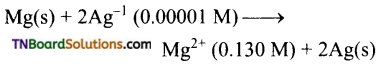Calculate the emf of the cell.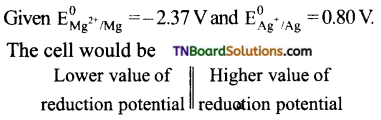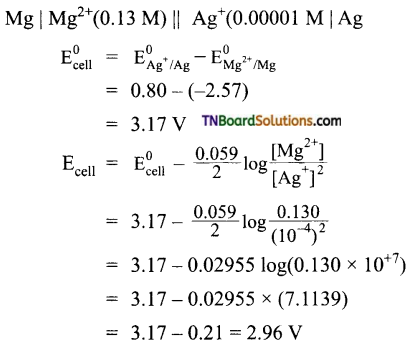Question 33.
Give the relationship between the free energy charge of the cell reaction and the emf of the cell.
ΔG° = -n FEcell

Question 34.
Give the relationship between the free energy change for the cell reaction and the equilibrium constant.
ΔG° = – 2.303 RT log Kc

Question 35.
(a) Calculate the standard free energy change and maximum work obtainable by a galvanic cell, where the cell reaction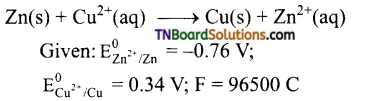(b) Also calculate the equilibrium constant for the reaction.
(a) The cell is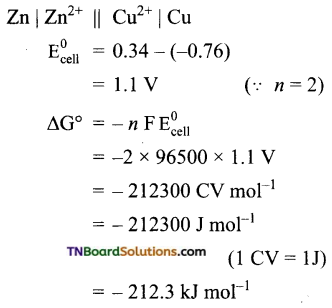Thus the maximum work that can be obtainable from the cell = 212.3 kJ mol-1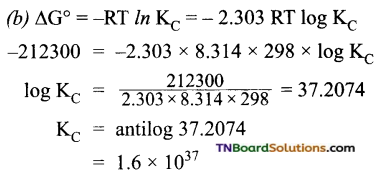Question 36.
Describe the nature of anode, cathode, the cell reaction, and salient features of the Leclanche cell.
Anode: Zinc container
Cathode: Graphite rod in contact with Mn02
Electrolyte: ammonium chloride and zinc chloride in water.
Emf of the cell is about 1.5 V.
Cell reaction:
Oxidation at anode:
Zn(s) → Zn2+(aq) + 2e … (1)
Reduction at cathode:
2NH4+(aq) + 2e → 2NH3(aq) + H2(g) … (2)
The hydrogen gas is oxidised to water by MnO2
H2(g) + 2MnO2(s) → Mn2O3(s) + H2O (l) …(3)
Equation (1) + (2) + (3) gives the overall redox reaction
Zn(s) + 2NH4+ (aq) + 2MnO2(s) → Zn2+(aq) + Mn2O3(s) + H2O (l) + 2NH3 ….. (4)
The ammonia produced at the cathode combines with Zn2+ to form a complex ion [Zn(NH3)4]2+(aq). As the reaction proceeds, the concentration of NH4+ will decrease and the aqueous NH3 will increase which lead to the decrease in the emf of cell.

Question 37.
Describe the nature of anode, cathode the cell reaction, and salient features of the mercury button cell.
Anode: Zinc amalgamated with mercury.
Cathode: HgO mixed with graphite.
Electrolyte: Paste of KOH and ZnO.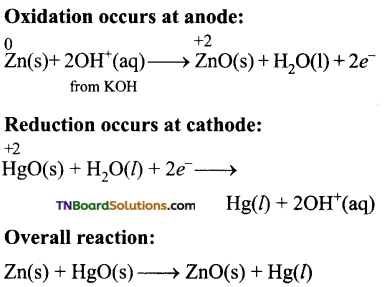Uses: It has a higher capacity and longer life. Used in pacemakers, electronic watches, cameras etc…

Question 38.
Explain the functioning of lead storage batteries.
[OR]
Explain the chemical reaction involved in the process of charging and recharging in a lead storage battery.
Electrolyte: 38% by mass of H2SO4 with density 1.2g / mL.
Oxidation occurs at the anode:
Pb(s) → Pb2+(aq) + 2e … (1)
The Pb2+ ions combine with SO4-2 to from PbSO4 precipitate.
Pb2+(aq) + SO42 (aq) → PbSO4(s) …….. (2)
Reduction occurs at the cathode:
PbO2(s) + 4H+(aq) + 2e → Pb2+(aq) + 2H2O (l) ……. (3)
The Pb2+ ions also combine with SO42- ions from sulphuric acid to form PbS04 precipitate.
Pb2+(aq) + SO42-(aq) → PbSO …… (4)
Overall reaction:
Equation (1) + (2) + (3) + (4) ⇒
Pb(s) + PbO2 (s) + 4H+(aq) + 2SO42-(aq) → 2PbSO4(s) + 2H2O (l)
The emf of a single cell is about 2 V. Usually six such cells are combined in series to produced 12 volt.
The emf of the cell depends on the concentration of H2SO4. AS the cell reaction uses SO22 ions, the concentration H2SO4 decreases. When the cell potential falls to about 1.8V, the cell has to be recharged.Question 39.
Discuss the nature of the anode, cathode, and the cell reaction of the Lithium-ion battery. How does it act as a secondary cell?
Anode: Porus graphite.
Cathode: transition metal oxide such as CoO2. Electrolyte: Lithium salt in an organic solvent At the anode oxidation occurs:
Li(s) → Li+(aq) + e
At the cathode reduction occurs:
Li+ + CoO2 (s) + e → Li CoO2 (s)
Overall reactions:
Li(s) + CoO2 → Li CoO2 (s)
Both electrodes allow Li+ ions to move in and out of their structures.
During discharge, the Li+ ions produced at the anode move towards the cathode through the non-aqueous electrolyte. When a potential greater than the emf produced by the cell is applied across the electrode, the cell reaction is reversed and now the Li+ ions move from cathode to anode where they become embedded on the porous electrode. This is known as intercalation.
Uses: Used in cellular phones, laptop computer digital camera, etc…

Question 40.
Mention the various methods of protecting metals from corrosion.
This can be achieved by the following methods.

1. Coating metal surface by paint.
2. Galvanizing by coating with another metal such as zinc, zinc is a stronger oxidizing agent than iron and hence it can be more easily corroded than iron, i.e., instead of iron, the zinc is oxidized.
3. Catholic protection: In this technique, unlike galvanizing the entire surface of the metal to be protected need not be covered with a protecting metal instead, metals such as Mg or zinc which is corroded more easily than iron can be used as a sacrificial anode and the iron material acts as a cathode. So iron is protected, but Mg or Zn is corroded.

Passivation: The metal is treated with strong oxidizing agents such as concentrated HNO3. As a result, a protective oxide layer is formed on the surface of the metal.
Alloy formation: The oxidizing tendency of iron can be reduced by forming its alloy with other more anodic metals.
eg: stainless steel – an alloy of Fe and Cr.1. In the electrolytic cell, the flow of electrons is from:
(a) cathode to anode in the solution
(b) cathode to the anode through external supply
(c) cathode to the anode through the internal supply
(d) anode to the cathode through the internal supply
(b)
Hint: In electrolytic cells, electrons enter the cathode and leave at the anode. Thus in the external supply, electrons flow from cathode to anode. In the solution, only ions flow and not the electrons.

2. Which of the statements about solutions of electrolytes is not correct?
(a) the conductivity of the solution depends upon the size of the ions.
(b) conductivity depends upon the viscosity of the solution.
(c) conductivity does not depend upon the solvation of ions present in the solution.
(d) the conductivity of the solution increases with temperature.
(c)
Hint: Greater the solvation of ions, lesser is the conductivity. Hence, (c) is an incorrect statement.

3. A dilute solution of Na2SO4 is electrolyzed using platinum electrodes. The products at the cathode and anode are:
(a) O2 and H2
(b) SO2, Na
(c) O2, Na
(d) S2O82-, H2
(a)
Hint: H20 is more readily reduced than Na+. It is more readily oxidised than S04 2. Hence, the electrode reactions are:
at cathode: 2H2O + 2e → H2 + 2OH
at anode: 2H2O → O2 + 4H+ + 4e.

4. The quantity of charge required to obtain one mole of aluminum from Al2O3 is:
(a) 1F
(b) 6F
(c) 3F
(d) 2F
(c)
Hint: Al2O3 → 2Al.
i.e., 2Al2O3 + 6e → 2Al or
Al2O3 + 3e → Al.
Hence, to obtain one mol of Al, the charge required is 3F.5. Electrolysis of dilute aqueous solution of NaCl solution was carried out by passing 10 milliampere current. The time required to liberate 0.01 mole of H2 gas at the cathode is (1 Faraday = 96500 C mol-1):
(a) 9.65 × 104 sec
(b) 19.3 × 104 sec
(c) 28.95 × 104 sec
(d) 38.6 × 104 sec
(b)
Hint: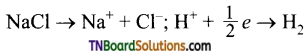Thus,
0.5 mol of H2 is liberated by IF = 96500 C 0.01 mol of H2 will be liberated by charge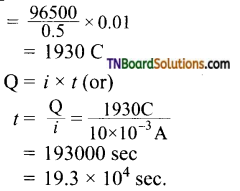6. Al2O3 is reduced by electrolysis at low potentials and high currents. If 4.0 × 104 amperes of current is passed through molten Al2O3 for 6 hours, what mass of aluminium is produced? (Assume 100% current efficiency. At mass of Al = 27 g mol-1):
(a) 8.1 × 104 g
(b) 2.4 × 105 g
(c) 1.3 × 104 g
(d) 9.0 × 103 g
(a)
Hint: Al3+ + 3e → Al
1 mol of Al = 27 g of Al is deposited by 3F or 3 × 96500 C of electricity.
Quantity of electricity passed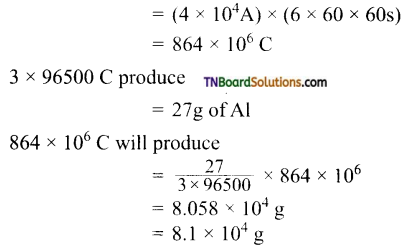7. What current is to be passed for 0.02 A for deposition of a certain weight of metal which is equal to electrochemical equivalent?
(a) 4 A
(b) 100 A
(c) 200 A
(d) 2 A
(a)
Hint: Electrochemical equivalent is the weight deposited by 1 coulomb.
Q = I × t
1 = I × 0.25 or I = 4A

8. Acertain current liberates 0.504 g of hydrogen in 2 hours. How many grams of oxygen can be liberated by the same current in the same time?
(a) 2.0 g
(b) 0.4 g
(c) 4.0 g
(d) 8.0 g
(c)
Hint: H2O → H2 + $$\frac{1}{2}$$ O2
Thus if one mole of H2 is liberated, O2 liberated = $$\frac{1}{2}$$ mole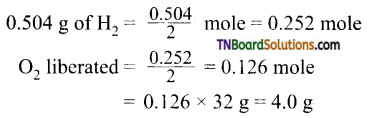9. Resistance of 0.2 M solution of an electrolyte is 50Ω. The specific conductance of the solution is 1.3 Sm-1. If the resistance of 0.4M solution of the same electrolyte is 260Ω the molar conductivity is:
(a) 6250 Sm2 mol-1
(b) 6.25 × 10-4 Sm2 mol-1
(c) 625 × 10-4 Sm2 mol-1
(d) 62.5 Sm2 mol-1
(b)
Hint:
specific conductance = observed conductance × cell constant
K = $$\frac{1}{R}$$ × cell constant
or cell constant = K × R
= 1.3 Sm-1 × 50Ω
= 65 m-1
For 0.4 M solution, specific conductance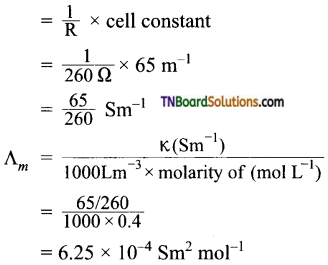10. An increase in equivalent conductance of a strong electrolyte with dilution is mainly due to:
(a) increase in the number of ions
(b) increase in ionic mobility
(c) 100% dissociation of electrolyte at normal dilution
(d) increase in both i.e., number of ions and ionic mobility of ion.
(b)
Hint: A strong electrolyte is completely ionized at all concentrations. Hence, the number of ions remains the same. However, on dilution, interionic forces decrease, and hence ionic mobility increases. Therefore, equivalent conductance increases.

11. In the electrolysis of which solution, OH ions are discharged in preference to Cl ions?
(a) dilute NaCl
(b) very dilute NaCl
(c) fused NaCl
(d) solid NaCl
(b)
Hint: In very dilute NaCl solution, the following reactions take place on electrolysis.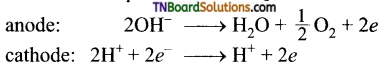12. The charge in coulombs on lg ion of N-3 is:
(a) 28.9 × 105 C
(b) 2.89 × 105 C
(c) 2890 C
(d) 28900 C
(b)
Hint: Charge on 1 gm ion of N-3
= 3 × 1.6 × 10-19 C
One g ion = 6.023 × 1023 ion
Charge on 1 gm ion of N-3
= 3 × 1.6 × 10-19 × 6.023 × 1023
= 2.89 × 105 C

13. A solution of copper sulfate is electrolyzed for 10 minutes with a current of 1.5 amperes. The mass of copper deposited at the cathode is: (atomic mass of Cu = 63.5 g mol-1)
(a) 29.6 g
(b) 0.296 g
(c) 2.96 g
(d) 1.564 g
(b)
Hint: Current = 1.5 amperes
Time = 10 minutes
= 10 × 60 = 600 s
Quantity of electricity passed = i × t
= 1.5 × 600 = 900 C
The reaction occurring at cathode is
Cu2+ + 2e → Cu
i.e., 2F or 2 × 96500 C deposit
= 1 mol = 63.5 g of Cu
900 C will deposit = $$\frac{63.5}{2 \times 96500}$$ × 900 = 0.296 g14. The limiting molar conductance of HCl, CH3COONa, and NaCl are respectively 425, 90, and 125 mho cm2 mol-1 at 25° C. The molar conductivity of 0.1 M CH3COOH solution is 7.8 mho cm2 mol-1 at the same temperature. The degree of dissociation of 0.1 M acetic acid at this temperature is:
(a) 0.1
(b) 0.02
(c) 0.15
(d) 0.03
(b)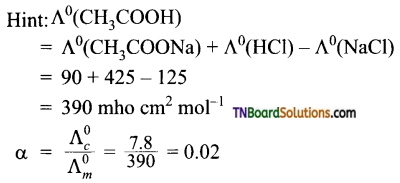15. Degree of dissociation of pure water is 1.9 × 10-9. Molar conductances of H+ and OH ions at infinite dilution are 200 S cm2 mol-1 and 350 Scm2 mol-1 respectively.
(a) 3.8 × 10-7 Scm2 mol-1
(b) 5.7 × 10-7 Scm2 mol-1
(c) 9.5 × 10-7 Scm2 mol-1
(d) 1.045 × 10-6 Scm2 mol-1
(b)
Hint: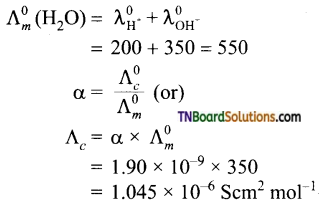16. When measured against a standard calomel electrode, an electrode is found to have a standard reduction potential of 0.100 V. If the standard reduction potential of the calomel electrode is 0.244 V, the standard reduction potential of the same electrode against the standard hydrogen electrode will be:
(a) – 0.144 V
(b) + 0.100 V
(c) – 0.344 V
(d) – 0.100 V
(b)
Hint: Standard electrode potential is a fixed quantity for a given electrode. Hence, its standard electrode potential against SHE will remain the same. Viz., (0.100 V). (emf of the cell formed will change)

17. On the basis of the following E° values, the strongest oxidising agent is: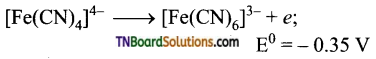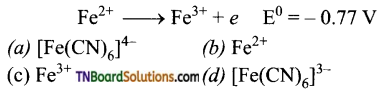(c)
Hint: The strongest oxidizing agent is a substance that is reduced move easily, i.e., it has the highest reduction potential. Reduction potentials of [Fe(CN)6]-3 and Fe3+ are 0.355 V and 0.77 V. Hence, Fe3+ is reduced more easily and hence is the strongest oxidizing agent.

18. Standard electrode potentials for Sn4+ / Sn2+ couple is +0.15 V and that for Cr3+/Cr couple is – 0.74 V. These two couples in their standard states are connected to make a cell. The cell potential will be:
(a) +1.83 V
(b) +1.19 V
(c) +0.89 V
(d) +0.18 V
(c)
Hint: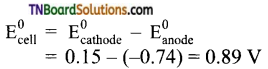19. Cr2O72- + I → I2 + Cr3+;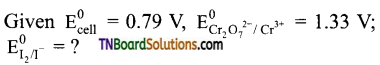(a) 0.54 V
(b) – 0.54 V
(c) +0.18 V
(d) -0.18 V
(a)
Hint: In the given reaction, I is oxidized to I2 and Cr2O72+ ions have been reduced to Cr3+. The cell is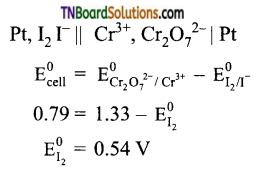20. Given the standard electrode potentials K+/K = -2.93 V; Ag+ / Ag = 0.80 V; Mg22+ / Hg = 0.79; Mg2+/Mg = -2.37 V; Cr2+/Cr = -0.74 V.
Arrange these metals in their increasing order of reducing power.
(a) Ag < Hg < Cr < Mg < K
(b) K < Mg < Cr < Hg < Ag
(c) Hg < Cr < Mg < K < Ag
(d) Mg < Cr < Hg < Ag < K
(a)
Hint: Lower the reduction potential, more easily it is oxidized and hence greater is its reducing power.

21. The reduction potential of hydrogen half cell will be negative if:
(a) pH2 = 1 atm and [H+] = 1.0 M
(b) pH2 = 2 atm and [H+] = 1.0 M
(c) pH2 = 2 atm and [H+] = 2.0 M
(d) pH2 = 1 atm and [H+] = 2.0 M
(b)
Hint: For hydrogen electrode,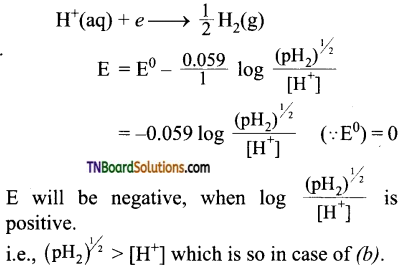22. 2Hg → Hg2+; E° = 0.855 V
Hg → Hg2+; E° = 0.799 V
Equilibrium constant for the reaction
Hg + Hg2+ ⇌ Hg22+ at 27° C is:
(a) 89
(b) 82.3
(c) 79
(d) none of these
(c)
Hint:
E° for the reaction = 0.855 – 0.799 = 0.056 V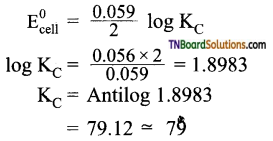23. Standard free energies of formation of (in kJ mol-1) at 298 K are -237.3, -394.2, and -8.2 for H2O(l), CO2(g), and pentane (g) respectively. The value of E°ell for the pentane-oxygen fuel cell is:
(a) 1.968 V
(b) 2.0968 V
(c) 1.0968 V
(d) 0.968 V